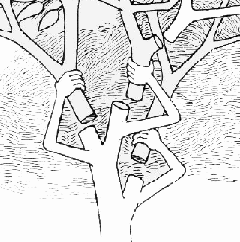## CS 250: Optimization Algorithms for Planar Graphs

Monday, Wednesday, Friday, 1:00-2:00
Instructor:
Location: CIT 477Planar graphs arise in applications such as road map navigation and logistics, graph drawing, and image processing. In this course, we study algorithmic techniques that exploit planarity in addressing classical problems, e.g. Traveling Salesperson, Shortest Paths,  and Maximum Flow

Prerequisite: CS 157 or equivalent (introductory algorithms)

## Focus

The focus this year is on algorithms for addressing and possibly implementing algorithms for logistics and planning problems in road maps. For example, see tsp.cs.brown.edu for a demo of an implementation of an approximation algorithm for traveling salesman in road maps.

## Textbook

Draft textbook chapters available at http://planarity.org (to be updated as the class proceeds).

## Work

Homeworks will be assigned once every week or two for the first three-fourths of the semester. During the last one-fourth of the semester, students will work on projects. In addition, there will likely be a midterm to ensure students have mastered the basics. Class participation will also affect students' grades.

## Outline

• Separators in trees
• Elementary graph theory
• Embedded graphs and duality
• Planar graphs and planar duality
• Maintaining a bounded-outdegree orientation
• Separators in planar graphs
• Primal-dual method for approximation
• Approximation algorithms for vertex-weighted Steiner trees and feedback vertex set
• Carvingwidth and branchwidth
• Optimization algorithms for graphs with bounded branchwidth
• Brenda Baker's method for approximation schemes
• Metric version of Baker's method, and approximation for {\em $k$-center}/{\em $r$-domination}
• Linear-time approximation scheme for traveling salesman problem
• Brick decomposition and approximation scheme for Steiner traveling salesman problem
• Approximation scheme for Steiner tree
• Prize-collecting clustering
• Approximation scheme for Steiner forest
• Bicriteria approximation scheme for bisection
• Maximum flow in directed $st$-planar graphs
• Shortest paths with nonnegative edge-lengths
• Dynamic-tree data structure
• Maximum flow in directed planar graphs
• Multiple-source shortest paths
• Maximum flow and multiple-source shortest paths in graphs with small weights
• Fast construction of brick decomposition
• Shortest paths in directed graphs with positive and negative edge-lengths
• Approximate distance oracle
• Fakcharoenphol-Rao priority queue
• Exact distance oracle
• Multiple-source multiple-sink maximum flow